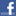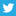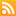# Scott Hanselman

## Flip the endian-ness of a long in C#

August 25, '06 Comments  Posted in Programming

Programming challenge:

Write me a function with this signature in C#:

public (unsafe?) long Reverse(long i, int bits)

...to flip the endian-ness (LSB/MSB) of a long, but just the # of significant bits specified.

Example, if the input is 376, with bits=11, the output is 244 (decimal, base 10).

376 = 00000101111000
244 = 00000011110100

Example, if the input is 900, with bits=11, the output is 270.

900 = 00001110000100
270 = 00000100001110

Example, if the input is 900, with bits=12, the output is 540.

900 = 00001110000100
540 = 00001000011100

Example, if the input is 154, with bits=4, the output is 5.

154 = 00000010011010
5   = 00000000000101

And make it FAST...;)

Scott Hanselman is a former professor, former Chief Architect in finance, now speaker, consultant, father, diabetic, and Microsoft employee. He is a failed stand-up comic, a cornrower, and a book author.Friday, August 25, 2006 6:55:06 AM UTC
I'd try something like this as the first guess:

public long Reverse(long i, int bits) {
i = ((i >> 1) & 0x5555555555555555) | ((i & 0x5555555555555555) << 1);
i = ((i >> 2) & 0x3333333333333333) | ((i & 0x3333333333333333) << 2);
i = ((i >> 4) & 0x0F0F0F0F0F0F0F0F) | ((i & 0x0F0F0F0F0F0F0F0F) << 4);
i = ((i >> 8) & 0x00FF00FF00FF00FF) | ((i & 0x00FF00FF00FF00FF) << 8);
i = ((i >> 16) & 0x0000FFFF0000FFFF) | ((i & 0x0000FFFF0000FFFF) << 16);
i = ( i >> 32 ) | ( i << 32);
return i >> (64 - bits);
}Nicholas Allen
Friday, August 25, 2006 7:03:58 AM UTC
I haven't tried this out, but here's my entry (divide and conquer, constant-time):

public long Reverse(long word, int bits)
{
ulong n = (ulong)word {{ (64-bits);
n = n }} 32 | n {{ 32;
n = n }} 0xf & 0x0000ffff | n {{ 0xf & 0xffff0000;
n = n }} 0x8 & 0x00ff00ff | n {{ 0x8 & 0xff00ff00;
n = n }} 0x4 & 0x0f0f0f0f | n {{ 0x4 & 0xf0f0f0f0;
n = n }} 0x2 & 0x33333333 | n {{ 0x2 & 0xcccccccc;
n = n }} 0x1 & 0x55555555 | n {{ 0x1 & 0xaaaaaaaa;
return (long) n | word & (-1 {{ bits);
}Wesner Moise
Friday, August 25, 2006 7:05:06 AM UTC
Nicely done, Dr. Nick.

I tried this:

long reverse(long data,int bits)
{
int i;
long l;
l = 0;
for(i = 0; i &lt; bits; i++)
{
l |= (long) (((data & (((long) 1)&lt;&lt;i)) ? 1:0)) &lt;&lt; (bits - 1 - i);
}
return l;
}

But got stuck in middle with the left shift not working the same in C# as it does in C++.

Then I headed in this general direction but got lost along the way:

public unsafe long Reverse(long i)
{
byte b1, b2, b3, b4;

b1 = (byte)(i & 255);
b2 = (byte)((i &gt;&gt; 8) & 255);
b3 = (byte)((i &gt;&gt; 16) & 255);
b4 = (byte)((i &gt;&gt; 24) & 255);

return (long)(((int)b1 &lt;&lt; 24) + ((int)b2 &lt;&lt; 16) + ((int)b3 &lt;&lt; 8) + b4);
}

You just whip yours out, or did you have it lying around?Scott Hanselman
Friday, August 25, 2006 7:06:36 AM UTC
Clever Wes, I'll test it and time it as well!Scott Hanselman
Friday, August 25, 2006 7:07:14 AM UTC
In the previous function, extend each of the constants to 64 bit length...Wesner Moise
Friday, August 25, 2006 7:10:38 AM UTC
The left shift operator should work the same in C# as in C++.
The right shift has two different behavior (arithmetic versus logical shift) depending on whether the type is signed or not.

(ulong) >>
(long) >>Wesner Moise
Friday, August 25, 2006 7:18:30 AM UTC
That reverse idea was classic on 16-bit micros. I just extended the pattern and fit it to your problem. I think Wes has the right idea with operating on ulong instead of long. I don't normally do a lot of bit flicking with C#.Nicholas Allen
Friday, August 25, 2006 11:50:22 AM UTC
So, which one was the fastest? :)Diego
Friday, August 25, 2006 3:34:30 PM UTC
The fastest way is going to use precomputation and table lookups.

http://jasonf-blog.blogspot.com/2006/08/hanselmans-endianess-converter.html

Friday, August 25, 2006 4:24:34 PM UTC
Scott! Do your own homework young man!haacked@gmail.com (Haacked)
Friday, August 25, 2006 6:32:34 PM UTC

http://aggregate.org/MAGIC/#Bit%20Reversal
Dilip
Friday, August 25, 2006 9:05:37 PM UTC
Not the most optimal solution, but another one none the less...

public long Reverse(long i, int bits)
{
long reversed = 0;
int j = 0;
for (j = 0; (j < (bits / 2)) && (j < 32); j++)
{
// Swap elements j, bits-j
reversed |= (((i >> (bits - j - 1)) & 0x1) << j);
reversed |= (((i >> j) & 0x1) << (bits - j - 1));
}
if (0 != (bits % 2))
{
// for an odd bits value, assign the middle value
reversed |= (((i >> (bits - j - 1)) & 0x1) << j);
}

return reversed;
}

I'd be interested in seeing the timing results...

-JoeJoe Pruitt
Friday, August 25, 2006 9:57:47 PM UTC
I went for the straight-forward

public static long Reverse(int i, int bits)
{
int rev = 0;
while (bits-- > 0)
{
rev <<= 1;
rev = rev + (i & 1);
i >>= 1;
}
return rev;
}

Yes, mine is O(N), but N is the value of bits, which is <= 32, while all the so-called O(1) solutions here are really O(ln N) where N is the number of bits in i. I would expect an insignificant speed difference among them.James Curran
Friday, August 25, 2006 10:36:17 PM UTC
BTW, this has nothing to do with Endian-ness, which is always byte-by-byte, not bit-by-bit.James Curran
Friday, August 25, 2006 10:40:25 PM UTC
James - heh! you're right. I'm just asking to flip the MSB and LSBs...the whole structure on it's ass.Scott Hanselman
Friday, August 25, 2006 11:08:40 PM UTC
public static long Lusix(long l, int bits)
{

long result;
long rval;
long calculatedRight=0;

// Get the block of bits
result = l & m.Right;
for (int i = 0; i < bits; i++)
{
// result = 543
rval = result >> (bits - (i + 1)); // if bits is 3 then rval=5

calculatedRight += (rval << i);

// Calculate new result
result = result - (rval << bits - (i + 1));

}

// Zero out the right part... and keep just the left part.

// Return the left part and the new calculated right part.
}

{
private long _left;
private long _right;

{
this._left = (long.MaxValue) << bits;
this._right = long.MaxValue >> (64 - (1 + bits));
}

public long Left
{
get { return _left; }
}

public long Right
{
get { return _right; }
}

}Ivan Terrence
Saturday, August 26, 2006 12:46:49 AM UTC
The looping solution works fine with shift operators, and this is the approach I tried first. I generally find that it's easy to become confused on complex operations, so I prefer to write my first approach with each step on individual lines for easier debugging (and to keep my mind straight). Once I prove the general solution works, I use ReSharper to inline the variables one at a time and add parantheses as necessary at each step.

public unsafe static long Reverse(long i, int bits)
{
long x = 0;

for(int bit = 0; bit < bits; bit++)
{
x |= ((i & (1 << bit)) == 0) ? 0 : (1 << (bits - bit - 1));
}

return x;
}

I was curious about the difference between this solution and Nicholas' solution, so I called each method 4,000 times in a loop. According to Performance Explorer using instrumented profiling, the looping solution requires 0.822978 seconds whereas Nicholas' solution requires 0.418179 seconds (roughly twice as fast).Lothan
Saturday, August 26, 2006 1:24:30 AM UTC
I smell a Hanselminutes topic: ways to instrument code and/or measure performance...

I tried timing all of the variations using the StopWatch class (starting it before the function call, and stopping it after, repeating for x iterations, and then averaging the ElapsedTicks. The results usually put Nicholas's solution first, but not consistently. Ivan's approach was consistently always last, though. :(

But, then out of curiosity, I commented out the Reverse() function calls, and didn't see any changes in my timings!!! That suggests some sort of Heisenberg phenomenon with my method.
Saturday, August 26, 2006 12:49:59 PM UTC
JasonF, table lookup is not always the fastest solution. The CPU can often perform computations faster than looking up memory.Wesner Moise
Saturday, August 26, 2006 4:45:40 PM UTC
Wesner: I came to realize that same conclusion as part of exploring this topic. Nicholas's algorithm above is consistently fast. It wasn't until I used a 16-bit lookup table that my algorithm would consistently beat his algorithm, but only at about 15-20% improvement in execution time at its best (this is, of course, assuming that the way I ended up capturing times turns out to be accurate).

An additional point of consideration: using 16-bit tables increased the memory requirements by a factor of 512 over the 8-bit table. So for the cost of 128+ KB of memory, you get a 15% improvement over Nicholas's algorithm that has no memory requirements. Memory is cheap, but in this case, would it make Scott's HDTV change channels any faster? Probably not.

The C# code from my trials is at the link that I posted above.
JasonF